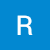Amazon 50 Best Seller Data Analysis using Plotly, Seaborn and Matplotlib.

import pandas as pd
import numpy as np
import matplotlib as plt
import seaborn as sns
import plotly.offline as pyo
import plotly.graph_objs as go
import plotly.express as px
%matplotlib inline

Students Performance in Exams.

#import libraries
# data processing libraries
import pandas as pd
import numpy as np
import matplotlib.pyplot as plt
import seaborn as sns

Python Linear Regression Tutorial machine learning in hindi.

# packages we will be using
import matplotlib.pyplot as plt
from sklearn import linear_model, metrics, model_selection
import numpy as np
import pandas as pdRohan sathe

data science & ml learner from heart & entrepreneur and designer